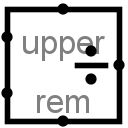#Teiler

 Bibliothek: Arithmetic Eingeführt: 2.0 Beta 22 Aussehen:## Verhalten

This component divides two values coming in via the west inputs and outputs the quotient on the east output. The component is designed so that it can be cascaded with other dividers to provide support a dividend with more bits than is possible with a single divider: The upper input provides the upper dataBits bits of the dividend (if it is specified at all), and the rem bits provide the remainder, which can be fed as the upper input into another divider.

If the divisor is 0, then no division is performed (i.e., the divisor is assumed to be 1).

The divider essentially performs unsigned division. That is, the remainder will always be between 0 and divisor-1. The quotient will always be an integer so that

quotient * divisor + remainder = dividend .
If, however, the quotient does not fit into dataBits bits, then only the lower dataBits bits will be reported. The component does not provide any method for accessing the upper dataBits bits.

If either of the operands contains some floating bits or some error bits, then the component's outputs will be either entirely floating or entirely error values.

## Pins

West edge, north end (input, bit width matches Data Bits attribute)
The lower dataBits bits of the dividend (that is, the first operand for the division).
West edge, south end (input, bit width matches Data Bits attribute)
The divisor (that is, the second operand for the division)
North edge, labeled upper (input, bit width matches Data Bits attribute)
The upper dataBits bits of the dividend (that is, the first operand for the division).
East edge (output, bit width matches Data Bits attribute)
The lower dataBits bits of the quotient, as specified above.
South edge, labeled rem (output, bit width matches Data Bits attribute)
The remainder of the division. This value will always be between 0 and divisor-1.

## Attribute

When the component is selected or being added, Alt-0 through Alt-9 alter its Data Bits attribute.

Data Bits
The bit width of the values to be divided and of the result.

Keines.

## Verhalten des Textwerkzeugs

Keines.

Zurück zur Bibliotheksreferenz